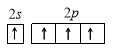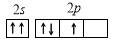## Chemistry Aptitude Quiz

IIT JEE exam which consists of JEE Main and JEE Advanced is one of the most important entrance exams for engineering aspirants. The exam is held for candidates who are aspiring to pursue a career in the field of engineering and technical studies. Chemistry is important because everything you do is chemistry! Even your body is made of chemicals. Chemical reactions occur when you breathe, eat, or just sit there reading. All matter is made of chemicals, so the importance of chemistry is that it's the study of everything..

Q1. REM is a unit of
•  Binding energy
•  Packing fraction
Solution
Part A

Q2.The possible sub-shells in n=3 energy shell are:
•  s,p,d
•  s,p,d,f
•  s,p
•  s only
Solution
Part A

Q3.  In a series of three steps in radioactive disintegration sequence starting with _88^228 Ra,the particles emitted are, successively, β^-,β^-and α-particles. The resulting product is an isotope of
•   _92 U
•   _90 Th
•   _88 Ra
•   _86 Rn
Solution
(c) _88^228 Ra□(→┬(- β) ) □(→┬( -β ) ) _90^228 X□(→┬( -α ) ) _88^224 Y
Q4.   The work function of a metal is 4.2 eV. If radiations of 2000 Å fall on the metal, then the kinetic energy of the fastest photoelectron is:
•  1.6×10^(-19)J
•  16×10^10J
•  3.2×10^(-19)J
•  6.4×10^(-10)J
Solution

(c)
KE = (Energy of radiation - Work function)
=(h×c/λ-4.2)
=((6.6×10^(-34) Js×3×10^8 m)/(2000×10^(-10) m))
-(4.2×1.602×10^(-19) J)
=(9.9×10^(-19) J)-(6.7×10^(-19) J)
=3.2×10^(-19) J
Q5.1 g of _79^200 Au (T_50=13 days)emits a β-particle forming a stable Hg atom. Hg formed at the end of 52 days is
•  0.0625 g
•  0.9375 g
•  0.7500 g
•  0.2500 g
Solution
(b)
Number of half-life =52/13=4
Au left after 52 days, C=C_0 (1/2)^n=1(1/2)^4=1/16
Hg formed =1-1/16=15/16=0.9375 g

Q6. If the threshold wavelength (λ_0) for ejection of electron from metal is 330 nm, then work function for the photoelectric emission is
•  1.2×10^(-18)J
•  1.2×10^(-20)J
• 6×10^(-29)J
•  6×10^(-12)J
Solution
(b)
Work function = Threshold energy =hv_0=hc/λ_o =(6.6×10^(-34) Js×3×10^8 m)/(330×10^(-9) m)=6.6×10^(-29) J

Q7.For which of the following electron distributions is ground state, the Pauli’s exclusion principle is violated?
••••Solution
Part C

Q8.The transition in He^⊕ ion that would have the same wavelength as the first Lyman line in hydrogen spectrum is
•  2→1
•  5→3
•  4→2
•  6→4
SolutionQ9.Aluminiu-25 decays by emitting a positron. The species immediately produced has
•  12p,13n,13e^-
•  13p,12n,13e^-
•  12p,13n,12e^-
•  14p,11n,14e^-
SolutionQ10. The reaction
〖 _1 D〗^2+〖 _1 T〗^3→ _1 He^2+〖 _0 n〗^1
Is an example of
•  Nuclear fission
•  Nuclear fusion
Solution
Part B#### Written by: AUTHORNAME

AUTHORDESCRIPTION## Want to know more

Please fill in the details below:

## Latest NEET Articles\$type=three\$c=3\$author=hide\$comment=hide\$rm=hide\$date=hide\$snippet=hide

Name

ltr
item
BEST NEET COACHING CENTER | BEST IIT JEE COACHING INSTITUTE | BEST NEET & IIT JEE COACHING: STRUCTURE OF ATOM Quiz-7
STRUCTURE OF ATOM Quiz-7
https://1.bp.blogspot.com/-3VY_xzOcPrk/YOgM6bMyXOI/AAAAAAAA38g/u6uZ88RsQWEKl2MrRXEWF1OkRVmp-bmZwCLcBGAsYHQ/s960/Quiz%2BImage%2B20%2B%252831%2529.jpg
https://1.bp.blogspot.com/-3VY_xzOcPrk/YOgM6bMyXOI/AAAAAAAA38g/u6uZ88RsQWEKl2MrRXEWF1OkRVmp-bmZwCLcBGAsYHQ/s72-c/Quiz%2BImage%2B20%2B%252831%2529.jpg
BEST NEET COACHING CENTER | BEST IIT JEE COACHING INSTITUTE | BEST NEET & IIT JEE COACHING
https://www.cleariitmedical.com/2021/07/structure-of-atom-quiz-7.html
https://www.cleariitmedical.com/
https://www.cleariitmedical.com/
https://www.cleariitmedical.com/2021/07/structure-of-atom-quiz-7.html
true
7783647550433378923
UTF-8

STAY CONNECTED